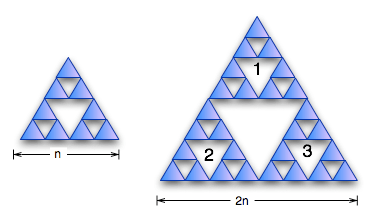# Fractal DimensionOne of the most fundamental properties of fractals that we’ve mostly avoided so far is the idea of dimension. I mentioned that one of the basic properties of fractals is that their Hausdorff dimension is
larger than their simple topological dimension. But so far, I haven’t explained how to figure out the
Hausdorff dimension of a fractal.

When we’re talking about fractals, notion of dimension is tricky. There are a variety of different
ways of defining the dimension of a fractal: there’s the Hausdorff dimension; the box-counting dimension; the correlation dimension; and a variety of others. I’m going to talk about the fractal dimension, which is
a simplification of the Hausdorff dimension. If you want to see the full technical definition of
the Hausdorff dimension, I wrote about it in one of my topology posts.

Starting from the basics: why do we need a different definition of dimension? There’s a nice, simple
definition of dimension used in geometry and topology. Why do we need to introduce this whole mess
of other measures of dimensionality?

Think about the Koch curve. Pick any two points on it. How long is the curve segment between them? It’s infinite. That means that it’s got an infinite amount of structure in the segment between the two points. But if it’s a curve, it’s one-dimensional. How can two points on a continuous 1-dimensional line segment be infinitely far apart? Clearly it’s possible, and yet it doesn’t jibe with our notion of how a one-dimensional thing should behave.

Think about the Sierpinski gasket. If you look at it, it seems to have a two-dimensional structure. But
if you work through the usual topological definition of dimension, it’s only got one dimension. Once again, the topological dimension doesn’t fit our intuition – it doesn’t really accurately describe the
apparent dimensional properties of the structure.

To fix this, we need some other notion of what dimension means. The fractal dimension is a measure
of how the complexity of the figure increases as it scales. The dimension is the exponent that
relates the scaling factor to the measure of the figure – scaledim=number of copies.

Let’s start with a line-segment. Double the length of the segment; how much have you increased the number of copies of the segment? You’ve doubled it. So increasing its size by a factor of 2 scales it up by two. That makes the line one dimensional.

Now, think about a square. Double the scale of the square – you’ve created 4 copies of it. Doubling scale creates 4 copies; 2dim=4, so the dimension is two. Now a cube: 2dim=8, so the dimension of the cube is three.

Good so far: this notion of dimension
produces results as we expect for simple regular shapes. Now let’s try it on fractals

Think about the Cantor set. To create a copy of the set, you need to triple its length (you need to add a blank space of size equal to the length of the original set, and then another copy of the original set).
So increasing the scale of the set by three creates two copies: 3dim=2; so dim = log32 = ln(2)/ln(3)=0.63.Now let’s look at the Sierpinksi gasket. If we double its size, we create three copies of it. So
its dimension can be calculated by 2dim=3. So dim=ln(3)/ln(2) = 1.58.One more: the Sierpinski carpet. To scale it up, we need eight copies to scale it by a factor of 3.
3dim=8; dim = ln(8)/ln(3)=1.89.

## 0 thoughts on “Fractal Dimension”

1.Andrew

How does the Hausdorff dimension differ from the box-count method? If I carefully box-count Sierpinski’s Gasket, should I get the same 1.585? Are there fractals which are better suited for box-count vs. Hausdorff or vise-versa? Can you use Hausdorff on the coast of England?
PS. tiny nit-pick shouldn’t the larger carpet be 3n instead of 3?

2.Brian

So, in application what does calculating the fractal dimension really say? For example (and like Andrew says above) can we do this calculation on natural fractals and compare them? Can you think of a hypothetical example where we would learn something by doing this?
thanks for all these posts….they are great!

3.Brent

Speaking of Menger Sponges… I urge you all to check this out.
http://www.theiff.org/oexhibits/paper06.html

4.Doug

This [fractals] may be a chaos game [Attractors / Dissipators]?
http://mathworld.wolfram.com/ChaosGame.html
A variation of Creation / Annihilation Transformations?

5.Jean-Philippe Poton

In reply to Andrew and Brian.
In most cases, the Hausdorff dimension differs from the Box-counting dimension and is smaller than it. They only coincide for some self-similar sets, which are not too irregular. In the case of Iterated Functions Systems, there is even a condition on the Similarities (sometimes called the “open set condition”) that ensures this equality.
The advantage of the Hausdorff dimension is that it is mathematically easier to manipulate, on the other hand, in a real-life case, the set under study is never simple enough to explicit a covering that would allow to calculate this dimension (furthermore, until proven otherwise, there is no fractals in nature, only objects or processes that can worthily be modelised as fractals, a fractal is an ideal object that is infinitely broken).
In particular, the coast of England is not self-similar, it is not even self-affine, what we know is that it can conveniently be modelised as a fractal. And so are many processes.
Some of the most interesting fractals though are random, the fractal dimension (which, in practice is mostly a Box-counting dimension or a derivation of it) is an interesting indicator of the entropy of a chaotic system, and in finance, we can for instance get some valuable insights about whether the system is trending or reversing,…